﻿ A Grey Approach for the Prediction of Supply Chain DemandPublications are Open
Access in this journal
Article Versions
Export Article
Cite this article
• Normal Style
• MLA Style
• APA Style
• Chicago Style
Research Article
Open Access Peer-reviewed

### A Grey Approach for the Prediction of Supply Chain Demand

Amanat Ur Rahman , Marzia Tuz Zahura
American Journal of Industrial Engineering. 2018, 5(1), 25-30. DOI: 10.12691/ajie-5-1-4
Published online: July 05, 2018

### Abstract

With the progress of technology and globalization, competition has risen and so has the need to optimize the Supply Chain. More enterprises are now focusing on supply chain efficiency in order to increase its profit margin and customer satisfaction. A part of the solution for increasing supply chain efficiency lies in the ability to make accurate forecast of demands, since, it interacts with multiple component of the supply chain network. Use of statistical, heuristics and machine learning algorithms is very common for future time series prediction, however, the accuracy of prediction by these models are significantly affected by the uncertainty, imprecision, and the size of source dataset. Grey theory has shown its effectiveness for its quick, brief and accurate prediction for vague, incomplete and imprecise data sets. In this paper, the grey one order one variable model GM (1,1) is applied for demand prediction in a case where the source data is brief and highly uncertain. The effectiveness of GM (1,1) is tested against one of the most commonly used and established forecasting method, exponential smoothing technique, for vague, imprecise and incomplete dataset. Based on the simulated results, the prediction accuracy of the grey prediction model has been observed to be a better fit than that of exponential smoothing technique. The average relative error (ARE) from the grey prediction model satisfies the level 2 of the accuracy scale and also achieving a mean relative simulation accuracy of 95.5%. Hence, based to the observed results, it can be established the GM (1,1) can be effectively used for any future time series prediction in such cases.

### 1. Introduction

Supply Chain efficiency has always been a primary concern for organizations and a top focus of research in academia. With the progress of technology and globalization competition has risen and so has the need to optimize the supply chain (SC). Since the early 1990s, many enterprises have implemented numerous initiatives in order to maximize the revenue and reduce cost 1. Supply chain management (SCM) involves everything from procurement of raw materials to manufacturing, distribution, customer service and finally after sales service 2. The profit level of the company is significantly dependent on the performance of the supply chain. Hence, there is a need for integration throughout the chain. One aspect of optimizing supply chain performance is to effectively predict the demand as its effect encompasses many of the components of SC, namely, inventory, transportation, distribution etc. Demand prediction is the process of prediction of future time series based on past data. The effectiveness of supply chain depends on primarily on the accuracy of prediction for product sales 3, 4, while the efficiency of the supply management optimization relies on the accuracy of forecast for the final product sales 3, 4, 5. Hence, the need for accurate and reliable prediction of future demand. Accurate forecasting allows companies to adjust to the changeable market, reduce cost of inventory, improve customer satisfaction and enhance competitiveness 6. However, demand is uncertain in most cases. Its irregularity, non-linearity, and randomness often hinders the accuracy of forecast.

A large number of forecasting methods have been used by researchers. Xia 4 mentions that these forecasting techniques can be classified into two categories: classical model and heuristics models. The classical models are generally based on mathematical and statistical algorithms. One of the commonly used and established classical model is exponential smoothing (ES) technique. The forecast is made based on the time series characteristics subjected to appropriate choice of smoothing constant 7. However, the choice of smoothing constant is hard to obtain due to lack of information or high uncertainty 8. The choice of the smoothing constant, as generally observed, is largely dependent on the analyst’s intuition and experience. Use of simple exponential smoothing (SES) is appropriate for a series that moves randomly above and below a constant mean and it has no trend and no seasonal patterns 9. Exponential smoothing has been vastly used in inventory demand forecasting 10. Its performance in forecasting is surprisingly well compared to more sophisticated approaches 10, 11, 12.

As opposed to the classical methods, modern heuristic approaches involve use of machine learning algorithms and evolutionary computations. Heuristic approaches include artificial neural network (ANN) 13, 14, fuzzy system analysis 15, 16 and also many hybrid models 17, 18. Although the algorithms are different, the objective is the same i.e. increasing the prediction accuracy. Prediction accuracy of both classical and machine learning techniques are significantly affected by the availability of sufficient source data and the uncertainty of these data.

Grey system theory, introduced originally by Deng in 1982 19, 20, is a multidisciplinary theory that has been widely used for solving vague, incomplete and imprecise dataset 19, 20, 21. In the field of information research, systems with completely unknown information are considered to be black, system with completely known information is regarded as white, and systems with partially known and partially unknown information are seen as grey 22. Application of grey theory is seen on system analysis, data processing, modelling, prediction, decision making and control 23. Prediction of any periodic evaluations of dataset can be done using grey prediction model as it does not have any prerequisite or restrictions on the source data 24, 25. Qu 26 used grey prediction model to predict mobile communication service income and achieved reasonably good accuracy compared to other nonlinear models. Samvedi 1 used grey prediction theory to predict supply chain during intermittent disruption. He argued that the grey model is a better fit when the disruption frequency is high. Grey prediction model has also been applied to predict supply chain performance resilience 27. Overall the application of the grey prediction model can be categorized as time-series prediction, calamity prediction, seasonal calamity prediction, topological forecasting and systematic forecasting 28. The one order one variable grey model GM (1,1) is the most widely used grey prediction model has a potency level of four i.e. it can predict with significant level of accuracy when the source data available in the time series is as low as four 23.

In this paper, grey prediction is made using GM (1,1). In order to benchmark the obtained prediction results, a comparison is made with an established and widely used forecasting model (SES). The rest of the paper is organized as follows. Section 2 discusses the traditional GM (1,1) and the exponential smoothing model. Section 3 discusses the steps in developing the mathematical model along with error analysis based on the empirical study. Finally, Section 4 concludes the paper.

### 2. Prediction Methodologies

2.1. Grey Prediction Model

GM (1,1) is the most commonly used grey prediction model for time series prediction. The letters GM stands for grey model and the “(1,1)” means one order one variable model. In the grey prediction model GM (1,1), the original data sequence is aggregated with the progression of time sequence. The aggregated data is used to construct a differential equation and upon solving the equation, using least square method, the prediction can be attained after performing inverse accumulated generation operator (AGO). Details of the grey prediction model is derived as follows 29.

2.1.1. Step 1: Establishment of the Original Data Sequence

Establish the data sequence from observed data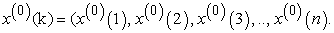2.1.2. Step 2: Generation of First Order Accumulated Generator Operator (1-AGO)

Generate the ﬁrst-order accumulated generating operation (1-AGO) sequence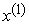is based on the original data sequence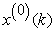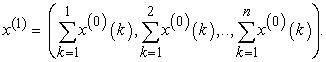This is done to reduce the randomness/noise in the raw data. These processed data becomes monotonously increasing function which complies with the solution of first order differential equation. As a result, the solution curve obtained from the differential equation is an approximate representation of 1-AGO data.

2.1.3. Step 3: Calculation of Background Value

Calculate the background value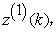by means of operation of on, the sequence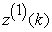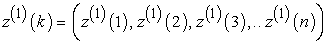Where,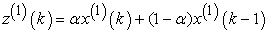and the generating coefficient α is usually given as 0.5.

2.1.4. Step 4: Construction of First-order Differential Equation

The first-order differential equation of GM (1,1) and its whitening equation is obtained, respectively, as follows: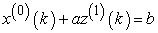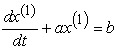Where ‘a’ and ‘b’ are the developing coefficient and grey input respectively.

2.1.5. Step 5: Solving the Differential Equation

Calculate development coefficient ‘a’ and grey input ‘b’. By using least square method parameter ‘a’ and ‘b’ can be obtained as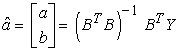Where,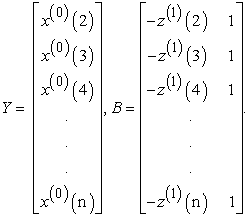2.1.6. Step 6: Solution of the Whitening Equation

The solution of the whitening equation is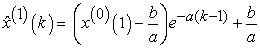Where,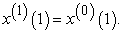2.1.7. Step 7: Generation of Prediction Sequence

The recovered data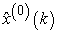can be retrieved by inverse accumulated generating operation (IAGO)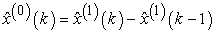Given k = 1,2,3,….,n.

Finally, the predictive value of sequence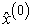is: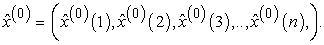2.2. Exponential Smoothing

The exponential smoothing is a time series prediction model that uses past data for future prediction. It is form of weighted moving average method that uses smoothing constant (α) to determine the amount of weight placed on the historical data sets. The value of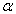ranges in between 0 and 1. The closer the value ofto 1, more weight is placed on recent past dataset. The basic simple exponential smoothing equation is as follows.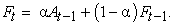Where,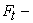Forecast for period t,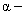Exponential smoothing constant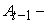Demand at time period t-1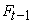- Forecast for time period t-1

Since the accuracy of the forecast using this model is greatly affected by the choice of value of the smoothing constant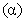, sensitivity analysis can be performed to determine the value of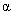such that the error is minimal.

### 3. Empirical Analysis

In order to demonstrate the effectiveness of the said methods, the sales data of a major pharmaceutical company of Bangladesh has been considered. The company name has been kept anonymous due reasons of confidentiality. The company is looking for a suitable and reliable prediction model considering the high uncertainty in the market. Management is looking to adopt new model provided that the prediction is relatively accurate. Using the sales data of 6 months, both the grey prediction model and exponential smoothing technique is applied for future time series prediction. The effectiveness of the model can be justified after performing accuracy and error analysis. The sales data of a newly launched product is shown in Table 1. Also, the pattern of data is seen in Figure 1.

3.1. Construction of the GM (1,1) model

The construction of the GM (1,1) model for demand prediction (Table 2) is discussed in the following steps.

I. The sales data from Table 1 are taken as input for the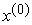and the cumulative sequence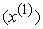is setup (Figure 2). The result is a monotonous increasing sequence that complies with the first order linear ordinary differential equation.

II. Test of the quasi-smoothness of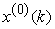and quasi-index patern ofis then checked. For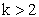, if, p(k) <.5 and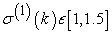the requirement of quasi-smoothness and quasi-index pattern is satisfied 30. As such, the observations from Table 2 confirms both the requirements are satisfied.

III. The background values are then calculated and acts as the input for matrix B while the original sequence acts as the input of matrix Y.

IV. The matrix is formed in order to solve the differential equation and obtain the result of the ‘a’ (developing coefficient) and ‘b’ (grey input). The solution can be estimated by least square method and obtain values of ‘a’ and ‘b’ are 0.021192189 and 17,354 respectively.

V. The values are fed into the whitening equation to get the corresponding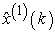.

VI. The prediction sequence is then retrieved by application of inverse accumulated generating operation (IAGO) as shown in Table 2.

• Figure 1. Sales data pattern

3.1.1. Accuracy of GM (1,1) Prediction

The error sequence,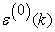, and the relative error,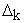, are found (Table 3) using the following formula.

Error Sequence: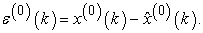Relative error: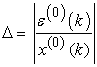Mean relative error,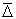, for the prediction model is found to be 0.04469 which satisfies the mean relative error requirement of 2nd level of accuracy scale 22. The mean relative simulation accuracy achieved is 0.95531 or 95.5 %.

A comparison figure for the actual sales data against the forecasted data is shown in Figure 3.

• Figure 3. Comparison between actual sales data and forecasted data
3.2. Construction of Exponential Smoothing Model

The construction of the exponential smoothing model is relatively simple. The accuracy of the model is largely dependent on the choice of the smoothing constant value (α). Due to unavailability of previous data starting forecast is assumed to be same as the actual sales (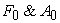equals the actual sales of first time period) in order to satisfy the exponential model equation. The steps in the model construction are as follows and the obtained results are represented in Table 4.

I. An initial forecast for the first period, which is the same as the actual sales, is made.

II. Following forecasts are made by choosing an arbitrary value of the smoothing constant (α).

III. For the obtained results, the mean absolute deviation is measured.

IV. Using SOLVER add-in in Excel, a sensitivity analysis is carried out to adjust the value of α, such that the mean absolute deviation is minimal.

V. The obtained value of α gives the minimal forecasting error and should be used for forecasting.

3.2.1. Accuracy of Exponential Smoothing Model

The respective error for the exponential smoothing technique,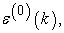for the time periods are shown in Table 5. Relative error,, and the mean relative error,, are found using the same mythologies as before.

The mean relative error,, for the exponential smoothing technique is found to be 0.08799533 which satisfies the average relative error requirement 3rd level of accuracy scale 22. The mean relative simulation accuracy obtained for the model is 0.912 or 91.2%. A comparison between the actual and forecasted data is shown in Figure 4.

• Figure 4. Comparison between actual and forecasted data
3.3. Comparison of Prediction Results

A comparison diagram between GM (1,1) prediction and exponential smoothing prediction against actual sales data is shown in Figure 5. Based on the results obtained, the GM (1,1) prediction is a better fit for the small, highly uncertain dataset. ES method seem to overshoot the prediction in almost all the time periods resulting in high degree of error. Accuracy obtained by this method is 91.2%. This could be due to the fact that the actual data seem to decrease over the time period. For any sudden fluctuation in the source data, the ES model would not be a good fit. On the other hand, GM (1,1) model was able to predict with 95.5% accuracy. This is because Grey theory bases its forecast on the aggregated source data rather than the discrete source dataset. Aggregated data reduces the randomness and thus greater prediction accuracy is achieved.

• Figure 5. Comparison of prediction results

### 4. Conclusion

With the increase in uncertainty and the complexity of modern day business, the need for an efficient supply chain is a must. An effective supply chain results in greater profitability and customer satisfaction for the company. Since forecasting is one of the most crucial component of supply chain the need for an accurate prediction model is mandatory. While many models and techniques exist, few can cope with the uncertainty in the source data. Also, the availability of data affects the accuracy.

In this paper, a local pharmaceutical company has been studied. The demand prediction of a newly launched product was the prime objective. Due availability of small data sets together with the high uncertainty in the local market use of machine learning algorithms and heuristics techniques deemed inappropriate. However, Grey theory deals with systems characterized by poor information, uncertainty, and imprecise data. Hence, in this paper, grey prediction model, GM (1,1), is implemented for the forecasting of demand along with one of the most established and commonly used prediction model, exponential smoothing technique. However, the accuracy of the prediction for the exponential smoothing technique was largely affected by the randomness, uncertainty, and availability of small source data whereas GM (1,1) is able to predict with significant level of accuracy. One of the reason for this is due to the fact that GM (1,1) uses the accumulated generation operation (AGO), which is one of the most important characteristics of grey theory, as it reduced the randomness of the data. The smoothness of the source data is checked by observing if changes in the data points are stable. This was done by checking the quasi-smoothness of the source data and the quasi-index pattern of the accumulated data. Hence satisfying the requirements for the use of GM (1,1) model. The prediction obtained a mean relative error of 0.04469 for the case of GM (1,1) as opposed to 0.087995 for ES. The mean relative error obtained for GM (1,1) and ES satisfies the second level and third level of accuracy scale. The mean relative prediction accuracy obtained for GM (1,1) is 95.5 % as opposed to 91.2% for ES.

Owing to the characteristic of the GM (1,1) model, the model provides an effective and efficient means of future time series prediction using only small amount of past data and thus a very appropriate model for prediction in such cases.

### References

  A. Samvedi and V. Jain, “A grey approach for forecasting in a supply chain during intermittentdisruptions,” Eng. Appl. Artif. Intell., vol. 26, no. 3, pp. 1044-1051, 2013. In article View Article  H. Balfaqih, Z. M. Nopiah, N. Saibani, and M. T. Al-Nory, “Review of supply chain performance measurement systems: 1998-2015,” Comput. Ind., vol. 82, pp. 135-150, 2016. In article View Article  S. C. Graves, D. B. Kletter, and W. B. Hetzel, “A dynamic model for requirements planning with application to supply chain optimization,” Oper. Res., vol. 46, no. 3-supplement-3, pp. S35-S49, 1998. In article View Article  M. Xia and W. K. Wong, “A seasonal discrete grey forecasting model for fashion retailing,” Knowledge-Based Syst., vol. 57, pp. 119-126, 2014. In article View Article  H.-S. Shih, E. S. Lee, S.-H. Chuang, and C.-C. Chen, “A forecasting decision on the sales volume of printers in Taiwan: An exploitation of the Analytic Network Process,” Comput. Math. with Appl., vol. 64, no. 6, pp. 1545-1556, 2012. In article View Article  L.-C. Hsu, “Applying the Grey prediction model to the global integrated circuit industry,” Technol. Forecast. Soc. Change, vol. 70, no. 6, pp. 563-574, Jul. 2003. In article View Article  R. Quintana and M. T. Leung, “Adaptive exponential smoothing versus conventional approaches for lumpy demand forecasting: case of production planning for a manufacturing line,” Int. J. Prod. Res., vol. 45, no. 21, pp. 4937-4957, 2007. In article View Article  R. Tsaur, “Forecasting by fuzzy double exponential smoothing model,” Int. J. Comput. Math., vol. 80, no. 11, pp. 1351-1361, 2003. In article View Article  V. Yorucu, “The analysis of forecasting performance by using time series data for two Mediterranean islands,” Rev. Soc. Econ. Bus. Stud., vol. 2, pp. 175-196, 2003. In article View Article  B. Billah, M. L. King, R. D. Snyder, and A. B. Koehler, “Exponential smoothing model selection for forecasting,” Int. J. Forecast., vol. 22, no. 2, pp. 239-247, 2006. In article View Article  S. Makridakis et al., “The accuracy of extrapolation (time series) methods: Results of a forecasting competition,” J. Forecast., vol. 1, no. 2, pp. 111-153, 1982. In article View Article  S. Makridakis and M. Hibon, “The M3-Competition: results, conclusions and implications,” Int. J. Forecast., vol. 16, no. 4, pp. 451-476, 2000. In article View Article  C. Hamzaçebi, “Improving artificial neural networks’ performance in seasonal time series forecasting,” Inf. Sci. (Ny)., vol. 178, no. 23, pp. 4550-4559, 2008. In article View Article  D. H. F. Yip, E. L. Hines, and W. W. H. Yu, “Application of artificial neural networks in sales forecasting,” in Neural Networks, 1997., International Conference on, 1997, vol. 4, pp. 2121-2124. In article View Article  S.-M. Chen, “Forecasting enrollments based on fuzzy time series,” Fuzzy sets Syst., vol. 81, no. 3, pp. 311-319, 1996. In article View Article  N. N. Karnik and J. M. Mendel, “Applications of type-2 fuzzy logic systems to forecasting of time-series,” Inf. Sci. (Ny)., vol. 120, no. 1-4, pp. 89-111, 1999. In article View Article  E. Hadavandi, H. Shavandi, and A. Ghanbari, “Integration of genetic fuzzy systems and artificial neural networks for stock price forecasting,” Knowledge-Based Syst., vol. 23, no. 8, pp. 800-808, 2010. In article View Article  G. P. Zhang, “Time series forecasting using a hybrid ARIMA and neural network model,” Neurocomputing, vol. 50, pp. 159-175, 2003. In article View Article  J.-L. Deng, “Control problems of grey systems.,” Sys. Contr. Lett., vol. 1, no. 5, pp. 288-294, 1982. In article View Article  D. Julong, “Introduction to grey system theory,” J. grey Syst., vol. 1, no. 1, pp. 1-24, 1989. In article View Article  S. Liu, J. Forrest, and Y. Yang, “A brief introduction to grey systems theory,” Grey Syst. Theory Appl., vol. 2, no. 2, pp. 89-104, 2012. In article View Article  S. F. Liu, Y. Yang, and J. Forrest, Grey Data Analysis. Springer, 2017. In article View Article  C. C. Hsu and C. Y. Chen, “Applications of improved grey prediction model for power demand forecasting,” Energy Convers. Manag., vol. 44, no. 14, pp. 2241-2249, 2003. In article View Article  C.-F. Tsai and S.-L. Lu, “Novel grey models for the trend forecast of Taiwan waste gas apparatus,” Int. J. Environ. Technol. Manag., vol. 18, no. 2, pp. 170-184, 2015. In article View Article  T. Xia, X. Jin, L. Xi, Y. Zhang, and J. Ni, “Operating load based real-time rolling grey forecasting for machine health prognosis in dynamic maintenance schedule,” J. Intell. Manuf., vol. 26, no. 2, pp. 269-280, 2015. In article View Article  P. Qu, “Mobile communication service income prediction method based on grey buffer operator theory,” Grey Syst. Theory Appl., vol. 4, no. 2, pp. 250-259, 2014. In article View Article  R. Rajesh, “Forecasting supply chain resilience performance using grey prediction,” Electron. Commer. Res. Appl., vol. 20, pp. 42-58, 2016. In article View Article  N. Xie and S. Liu, “Discrete grey forecasting model and its optimization,” Appl. Math. Model., vol. 33, no. 2, pp. 1173-1186, 2009. In article View Article  L.-C. Hsu, “Using improved grey forecasting models to forecast the output of opto-electronics industry,” Expert Syst. Appl., vol. 38, no. 11, pp. 13879-13885, Oct. 2011. In article View Article  C. Liu, T. Shu, S. Chen, S. Wang, K. K. Lai, and L. Gan, “An improved grey neural network model for predicting transportation disruptions,” Expert Syst. Appl., vol. 45, pp. 331-340, 2016. In article View Article

Published with license by Science and Education Publishing, Copyright © 2018 Amanat Ur Rahman and Marzia Tuz ZahuraThis work is licensed under a Creative Commons Attribution 4.0 International License. To view a copy of this license, visit http://creativecommons.org/licenses/by/4.0/

### Cite this article:

##### Normal Style
Amanat Ur Rahman, Marzia Tuz Zahura. A Grey Approach for the Prediction of Supply Chain Demand. American Journal of Industrial Engineering. Vol. 5, No. 1, 2018, pp 25-30. http://pubs.sciepub.com/ajie/5/1/4
##### MLA Style
Rahman, Amanat Ur, and Marzia Tuz Zahura. "A Grey Approach for the Prediction of Supply Chain Demand." American Journal of Industrial Engineering 5.1 (2018): 25-30.
##### APA Style
Rahman, A. U. , & Zahura, M. T. (2018). A Grey Approach for the Prediction of Supply Chain Demand. American Journal of Industrial Engineering, 5(1), 25-30.
##### Chicago Style
Rahman, Amanat Ur, and Marzia Tuz Zahura. "A Grey Approach for the Prediction of Supply Chain Demand." American Journal of Industrial Engineering 5, no. 1 (2018): 25-30.